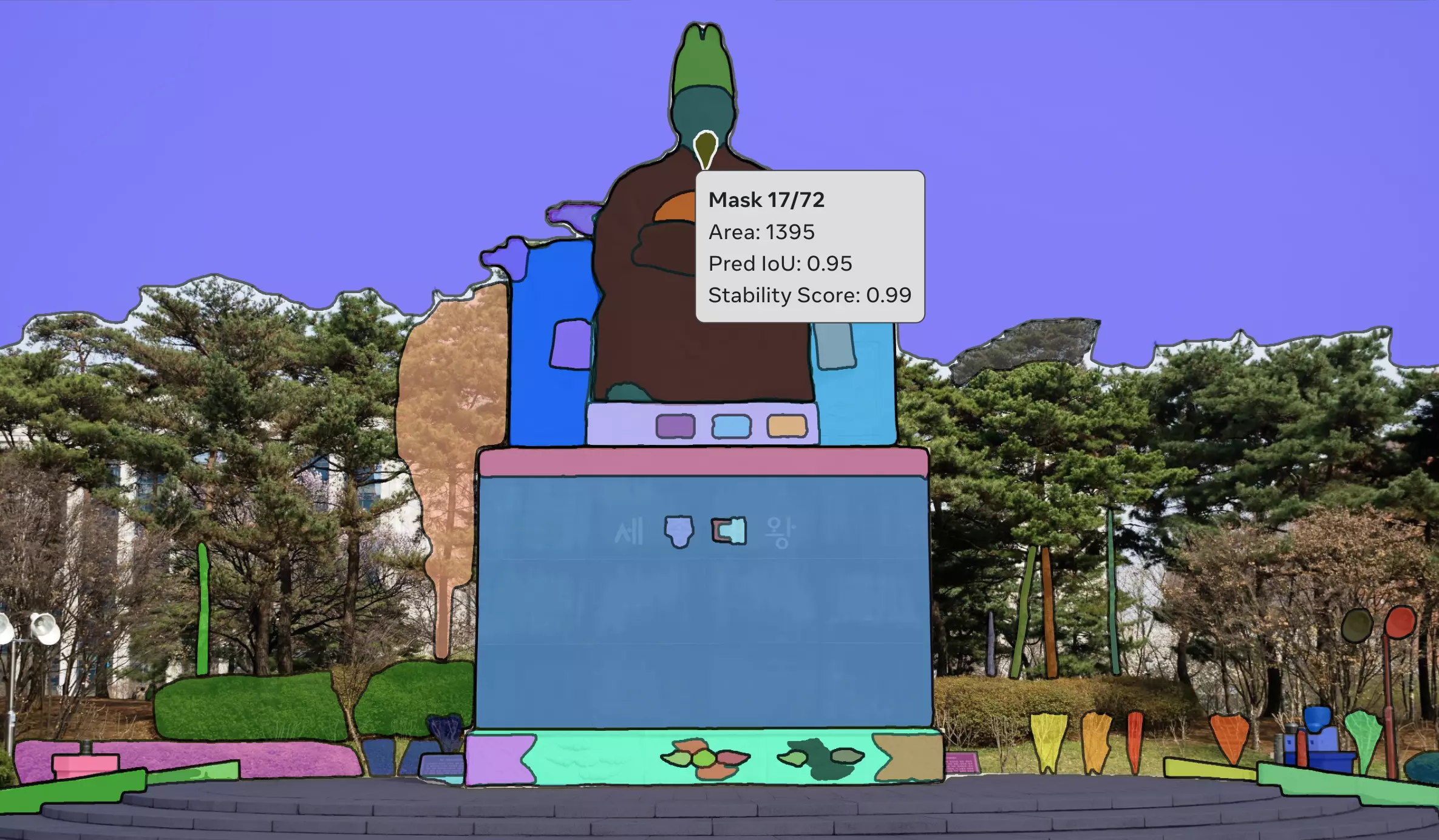Learning

# Segment Anything TorchServe deployment with Code

## Tutorial for a Segment Anything Torchserve deployment with code

2023
.
06
.
07
byLet's take a look at deploying the Segment Anything model with Torchserve with code. This post will be code-centric, so if you're interested in learning more about Torchserve, we recommend reading our Torchserve series on our blog. Let's get started. ‍

## Write a Torchserve handler

Let's write the config.properties and handler.py that we'll need for inferencing.

The address port in config.properties will set up port forwarding for future docker runs so that we can infer outside of docker. The gpu_id and batch_size are values that go into the properties:dict variable in handler.py.

``````# config.properties
gpu_id=0
number_of_gpu=0
batch_size=1
model_store=.
default_workers_per_model=1
``````

The basic code structure of handler.py is the same as the example code provided by Torchserve. However, we've added the process of base64 encoding the inference result in post-processing. It's fine to return it as a torch.Tensor or np.array, but we'll export it as a string type for convenient communication later.

``````# handler.py
import io
import os
import cv2
import torch
import base64
import pickle
import logging
import requests
import numpy as np
from PIL import Image
from ts.torch_handler.base_handler import BaseHandler
from segment_anything import sam_model_registry, SamPredictor

logger = logging.getLogger(__name__)

class SegmentAnythingHandler(BaseHandler):
def __init__(self):
super(SegmentAnythingHandler, self).__init__()
self.initialized = False

def initialize(self, ctx):
self.manifest = ctx.manifest
properties = ctx.system_properties
model_dir = properties.get("model_dir")
serialized_file = self.manifest["model"]["serializedFile"]
model_pt_path = os.path.join(model_dir, serialized_file)
self.device = torch.device(f"cuda:{properties['gpu_id']}" if torch.cuda.is_available() else "cpu")
sam = sam_model_registry[serialized_file.replace('.pth', '')](checkpoint=model_pt_path)
sam.to(self.device)
self.predictor = SamPredictor(sam)

logger.debug("Model from path {0} loaded successfully".format(model_dir))
self.initialized = True

def handle(self, data, ctx):
image_path = data.get("data")
if image_path is None:
image_path = requests.get(data.get("body")["dataUrl"]).content
image = Image.open(io.BytesIO(image_path))
image = cv2.cvtColor(np.array(image), cv2.COLOR_RGB2BGR)

self.predictor.set_image(image)
result_dict = {'original_size': self.predictor.original_size,
'input_size': self.predictor.input_size,
'features': post_process(self.predictor.features)}
return [base64.b64encode(pickle.dumps(result_dict)).decode('utf8')]
``````

Now that we're ready to deploy our model, let's create an inference environment using docker.

## Building a Segment Anything Docker Environment

If you're not using Docker, you can jump right into deploying the model.

First, let's create a DockerFile. You can choose any version of the base image, and we'll install the jdk since you'll need it to use Torchserve. Finally, we'll install the necessary libraries. You can create a requirements.txt file and install it with RUN pip install -r requirements.txt if you're comfortable.

``````FROM pytorch/pytorch:1.13.1-cuda11.6-cudnn8-runtime

WORKDIR /workspace
RUN mkdir datahunt_segment_anything

# Install OpenJDK-11
RUN apt-get update && \
apt-get install -y git && \
apt-get install -y openjdk-11-jre-headless && \
apt-get clean;

RUN apt-get -y install libgl1-mesa-glx && \
apt-get install -y curl;

RUN pip install opencv-python matplotlib onnxruntime onnx
RUN pip install torchserve torch-model-archiver torch-workflow-archiver nvgpu validators tensorflow-cpu
``````

Now let's build it.

``````docker build -t segment-anything:v1 .
``````

Next, we'll create a container and set up ports, and if necessary, set up volume mounting so we can see what we're doing locally in real time. But since we're going to be using the finished code, we won't set it up. -p 8070 is the port of the inference_address we set in config.properties above.

``````docker run -it --gpus all --name segment-anything-v1 -p 8070:8070/tcp segment-anything:v1 /bin/bash
``````

See that you're connected to the container? Now that we're all set for deployment, let's create a .mar file and run Torchserve.

## Deploying the Segment Anything model

The current working directory is /workspace/datahunt_segment_anything, and the internal folder structure is as follows.

Here's the script for deployment, you can run it one by one, but I'm going to write it as a shell script for future iterations. Since we're going to be using it for redistribution, I've added code to shut down the server if it's running, and delete any existing MARs that were created. If you don't need to do that, feel free to delete them.

``````#!/bin/bash

torchserve --stop

version=1.0
model_name="SAM"
model_path='../model/sam_vit_l.pth'

if [ -e \${model_name}.mar ]; then
rm \${model_name}.mar
echo 'Removed existing model archive.'
fi

# Create mar file
torch-model-archiver --model-name \${model_name} --version \${version} --serialized-file \${model_path} --handler "handler.py"

# Depolyment
torchserve --start --model-store . --models \${model_name}.mar --ts-config ./config.properties
``````

Now run the torchserve_deploy.sh file we wrote above, and the model deployment is complete. Let's infer the model. Let's prepare the desired sample and run it as shown below.

``````curl -X POST "http://127.0.0.1:8070/predictions/SAM/1.0" -T "test/sample/sample.jpg"
``````

Then you can see the result is output in the format you set in handler.py, as shown below.

## Segment Anything Promptable Task Reasoning Test

This is the process of fetching the pre-picked embedding results and proceeding with the Promptable Task. I used pre-existing values for the point and bounding box coordinates. I used Python code here, but the Github Demo has code for reasoning in other languages.

``````import cv2
import json
import torch
import base64
import pickle
import numpy as np
import matplotlib.pyplot as plt
from argparse import ArgumentParser
from segment_anything import sam_model_registry, SamPredictor

if random_color:
color = np.concatenate([np.random.random(3), np.array([0.6])], axis=0)
else:
color = np.array([30 / 255, 144 / 255, 255 / 255, 0.6])
plt.show()

if __name__ == '__main__':
parser = ArgumentParser()
args = parser.parse_args()

box_coords = np.array([198, 1369, 1369, 2665])
point_labels = np.array([1, 1, 1])
point_coords = np.array([[1000, 1700], [1200, 1500], [1300, 1700]])

image = cv2.cvtColor(image), cv2.COLOR_BGR2RGB)

device = torch.device(args.device)

sam = sam_model_registry[args.model_type](checkpoint=args.checkpoint)
sam.to(device=device)
predictor = SamPredictor(sam)

predictor.is_image_set = True
predictor.input_size = input_dict['input_size']
predictor.original_size = input_dict['original_size']
predictor.features = input_dict['features'].to(device)